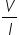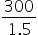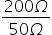Physics
Easy

Question

# Resistors, each of 50 Ω, are connected in a circuit in series. It gives a current of 1.5 A when connected across a 300 V supply. How many resistors are connected in series?

## 3456Hint:

## The correct answer is: 4

### 4 resistors are connected in series. The resistors in each series bear a voltage shared equally when it is supplied by a constant current voltage. The number of resistors determines the resistances in each series. Applying Ohm's law, V=IR R=== 200 Ω Let, x be the number of resistors in series, Thus, x== 4 Therefore, a series combination of 4 resistors gives the current of 1.5 A when connected across a 300 V supply.#### With Turito Foundation.#### Get an Expert Advice From Turito.